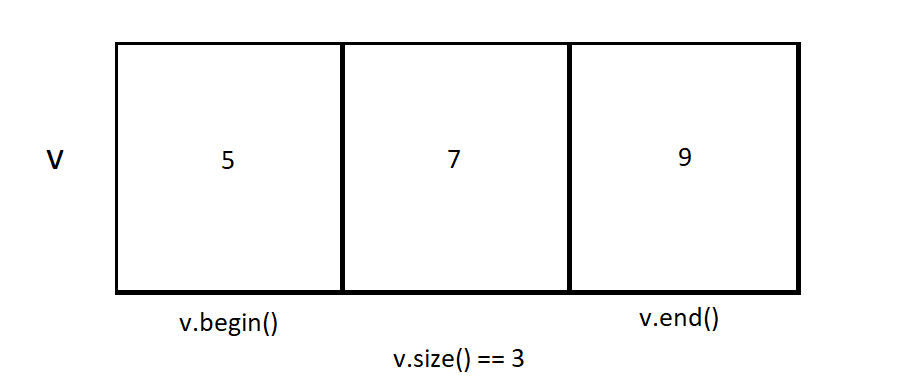×

Search anything:

# vector::size() vs vector::capacity()

#### C++Get this book -> Problems on Array: For Interviews and Competitive Programming

In this article, we will explore and contrast the size() and capacity() functions of the vector class in C++.

1. Introduction to vector::size()
2. vector::size() example
3. Introduction to vector::capacity()
4. vector::capacity() examples
5. Difference between vector::size() and vector::capacity()
6. Example using both functions
7. Time complexity of vector::size() and vector::capacity()

## Introduction to vector::size()

The size() member function of the vector class returns the number of elements in a vector. Here is the function's header:

``````size_type size() const noexcept;
``````

Note that "size_type" is the return type. This simply makes the type of number returned guaranteed to be adequate for covering the size of the vector.

The size() function is one of the more commonly used functions in the vector class since finding the number of elements contained in a vector is often necessary.

## vector::size() example

Here is a short program that demonstrates the vector::size() member function:

``````#include <iostream>
#include <vector>
using namespace std;
int main() {
vector<int> v;
v.push_back(5);
v.push_back(7);
v.push_back(9); //v == {5, 7, 9}, so v.size() == 3
for (int i : v) {
cout<<i<<" ";
}
cout<<"\n"<<"The size of the vector is "<<v.size();
return 0;
}
``````

Output:

``````5 7 9
The size of the vector is 3
``````

In this example, three elements (5, 7, and 9) were added to the vector. Since there were 3 elements total in the vector, when the size() function was called on the vector, it returned 3.## Introduction to vector::capacity()

The capacity() member function of the vector class returns the number of elements the vector currently has allocated space for. The capacity() function takes no parameters. Here is the function's header:

``````size_type capacity() const noexcept;
``````

Similar to size(), the return type is size_type. Note that capacity() does not return the absolute upper limit on the number of elements the vector can hold, but rather the limit on the number of elements can hold with the current amount of memory allocated. More memory can be allocated for the vector both automatically and manually. The absolute maximum amount of elements the vector can hold can be found from vector::max_size() instead.

## vector::capacity() examples

Here is a short program that demonstrates the vector::capacity() member function:

``````#include <iostream>
#include <vector>
using namespace std;
int main() {
vector<int> v;
v.push_back(5);
v.push_back(7);
v.push_back(9);
cout<<"The capacity of the vector is "<<v.capacity()<<endl;
return 0;
}
``````

Output:

``````The capacity of the vector is 4
``````

Note that even though the vector's size was 3, the capacity was 4. This difference is explained by the fact that the size member function's output depends on the amount of elements in the vector, but the capacity member function depends on the amount of memory allocated for the vector and how much memory is needed to store each element.

Here is another short program that uses the capacity() member function:

``````#include <iostream>
#include <vector>
using namespace std;
int main() {
vector<int> v;
for (int i = 0; i < 5; i++) v.push_back(i);
//capacity of the vector automatically increased when 2 more elements were added
cout<<"The capacity of the vector is "<<v.capacity();
return 0;
}
``````

Output:

``````The capacity of the vector is 8
``````

## Difference between vector::size() and vector::capacity()

The key difference between vector::size() and vector::capacity() is that while vector::size() returns the number of elements the vector contains, vector::capacity() returns the number of elements the vector currently has enough memory allocated to hold. vector::capacity() is always greater than or equal to vector::size()

Another difference is that you can change the capacity of a vector without actually changing what the vector contains (using vector::reserve() for example), but because the size() member function returns the number of elements, vector::size() can only change if elements are added to or removed from the vector.

Both vector::size() and vector::capacity() are useful and have practical applications. The size() method is commonly used during iterations via loops as well as other operations involving processing the elements of a vector. The capacity() method and its underlying concept are often used to debug and optimize the performance because the capacity of a vector can be changed so that is exactly large enough to hold what it needs to. This prevents memory from being wasted as it otherwise might have been if the capacity of the vector was ignored. It is worthwhile to keep the capacity of a vector in mind.

## Example using both functions

Here is a short program that uses both vector::size() and vector::capacity().

``````#include <iostream>
#include <vector>
using namespace std;
int main() {
vector<int> v; // v.size() == 0, and v.capacity() == 0
v.reserve(10); // v.size() == 0, and v.capacity == 10
for (int i = 0; i < 15; i++) v.push_back(i); //v.size() == 15
cout<<"The size of the vector now is "<<v.size()<<endl;
cout<<"The capacity of the vector is now "<<v.capacity()<<endl;
return 0;
}
``````

Output:

``````The size of the vector now is 15
The capacity of the vector is now 20
``````

Note that in the example, the size of the vector only changed when the number of elements changed, but the capacity of the vector was changed both manually using vector::reserve() and automatically when the capacity was insufficient for the size. As a reminder, the size() and capacity() functions do not always return the same value.

## Time complexity of vector::size() and vector::capacity()

The time complexity of vector::size() is O(1) since no matter the size of the vector, the only operation done by the function is returning the number of elements the vector currently contains.

Similarly, the time complexity of vector::capacity() is O(1) since no matter the size of the vector, the only operation done by the function is returning the number of elements the vector currently has enough memory allocated to contain.

With this article at OpenGenus, you should have the complete idea of vector::size() and vector::capacity() in C++ Programming Language.